Differential Equations IOn a cold day, it doesn't take long for a cup of coffee to become tepid; if our refrigerator breaks, our frozen items tend to warm up. These day-to-day experiences result from heat moving about, a topic that greatly interested Sir Isaac Newton.

Newton and his various laws will crop up from time to time in our course. He's particularly famous for inventing calculus and for his rules of motion, but he also discovered a law for cooling:

An object's temperature changes at a rate proportional to the difference between it and the surrounding temperature.

This will be our starting point for a quick pass through the essential ideas of differential equations. No experience with physics is required; just a little single-variable calculus and a dash of vector arithmetic will do.

Our First Equation

There are three steps in applying differential equations to the study of the natural world:

• Step 1: Come up with a differential equation using experiment-based principles.
• Step 2: Analyze the differential equation to make sure it's realistic.
• Step 3: If possible, solve the equation. If not, settle for what was learned in step 2.

Our aim is to use these steps to track the temperature $T$ of an object through time $t$ if it starts out cooler or warmer than the air around it.

According to step 1, we need to cook up a differential equation based on some physical principle. Sir Isaac Newton gives us exactly what we need:“An object's temperature changes at a rate proportional to the difference between it and the surrounding temperature.”

If the surrounding air has temperature $T_{\text{air}},$ which is a constant, what differential equation can we infer for $T(t)$ from Newton's law of cooling? In all options, $k$ is a constant.

Our First EquationDifferential equations modeling the real-world are usually built on principles discovered by scientists through careful experiments.

So, realistically, there's a step 0: be a scientist and gather empirical data! But we'll just reap the benefits of centuries of experiment and jump to step 1, which is what we did to find $\frac{dT}{dt} = k \big[ T(t) - T_{\text{air}} \big].$ Now in the second step, we need to critically analyze this equation. In other words, we're going to make sure it's a realistic model before going to the trouble of solving it.

We don't doubt Newton's law, but we don't know anything about $k$ other than it's constant. For the wrong choice of $k,$ our equation could predict something unrealistic for $T(t).$

For what condition on $k$ does the differential equation match our intuition about the real world?

Hint: Think about different cases for the initial temperature $T(0)$ and what the equation implies about these scenarios.

Our First Equation

We'll learn many techniques for solving differential equations in our course, but it sometimes happens that an equation is just too difficult to solve. That doesn't mean we give up and go home; we do what we can to glean as much information as possible from the equation itself.

So, instead of pressing on to step 3, let's stick with step 2 for a while and gather some qualitative information about our mystery function $T(t).$

Let's start with a fact that's true for all equations in our course: solution curves never cross!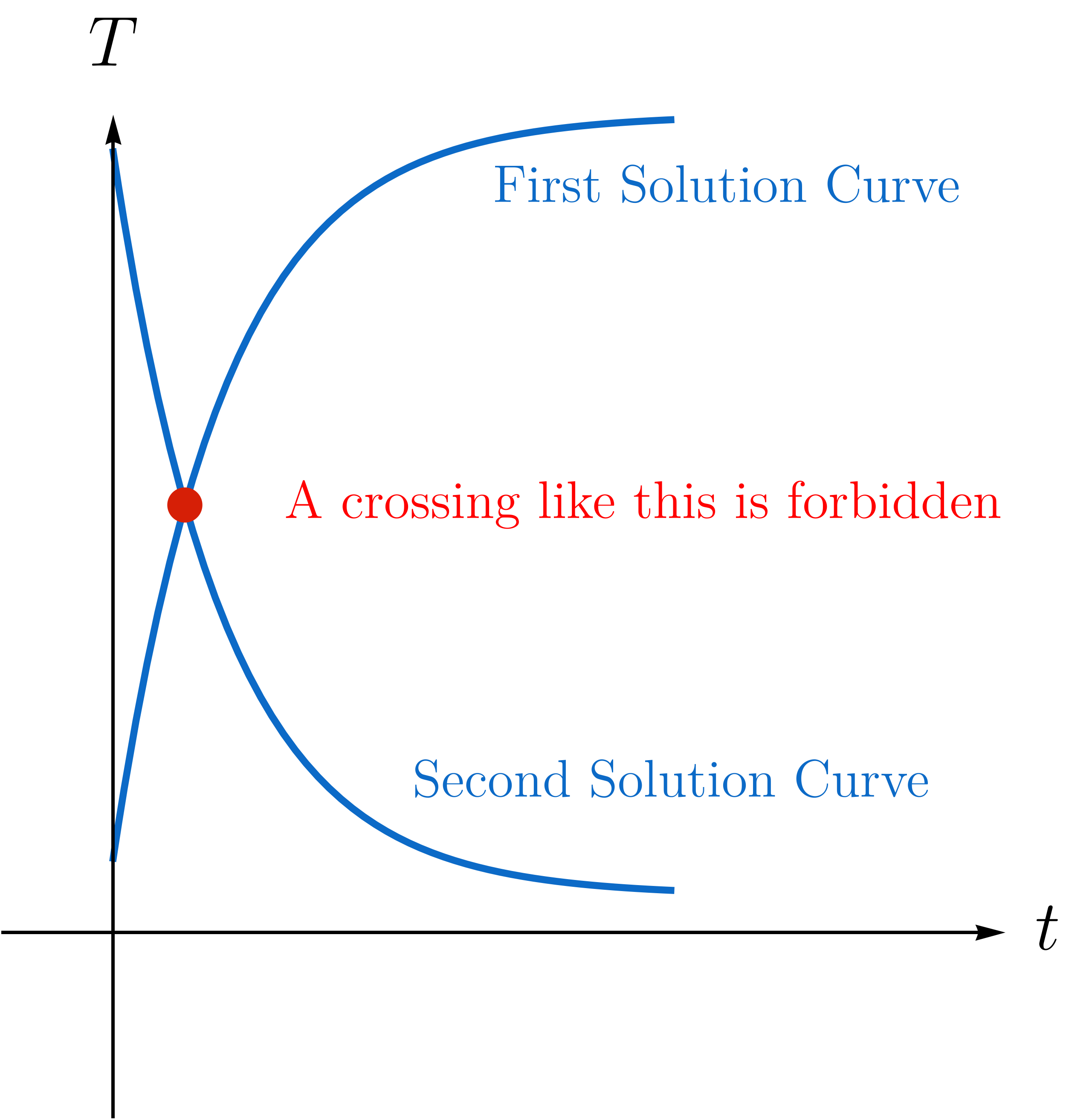We'd have some serious problems to deal with if solutions could cross. Take a cup of coffee, for instance. If we start measuring its temperature at the moment two different curves intersect, we couldn't predict its future: the real $T(t)$ would follow one of the two curves, but we couldn't tell which one!

If we take it for granted that solution curves never cross, what can we say about $T(t)$ from $\frac{dT}{dt} = k \big[ T(t) - T_{\text{air}} \big]?$ More than one option may be true!

Our First Equation

Select one or more

But how can $T(t)$ always increase when $T(0) < T_{\text{air}}$ and not cross over $T_{\text{air}} ?$ The answer lies in the concavity of $T(t),$ which measures the “bendiness” of its graph.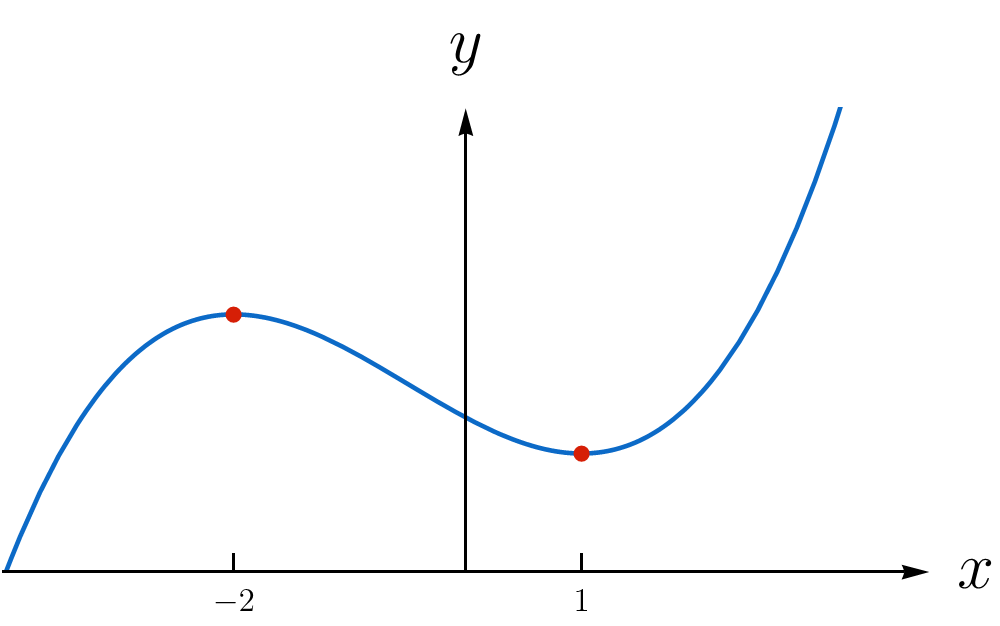If $y=f(x),$ the sign of $f''(x)$ determines if the best-fit parabola opens up/down.

Concavity is tied to the second derivative since it controls the shape of the best-fit parabola at a point: if $T''(t) > 0,$ the graph is concave up, while $T''(t) < 0$ means it's concave down.

Starting from $T'(t) = k \big[ T(t) - T_{\text{air}}\big],$ compute the second derivative $T''(t)$ and select from the options all true statements about the concavity of $T(t).$

Our First Equation

Select one or more

Let's summarize what we've found without solving the equation $T'(t) = k \big[T(t)-T_{\text{air}} \big]$:

If $T(0) > T_{\text{air}}$ $\big(T(0) < T_{\text{air}}\big),$ the function $T(t)$ is strictly decreasing (increasing) and its graph is always concave up (down).

So the graph of $T(t)$ depends on the initial condition $T(0),$ which isn't surprising given what we uncovered in the last unit. Each of the four plots below shows two graphs that could be potential solution curves, one with initial condition above $T_{\text{air}},$ the other below.

Select the only option that displays correct graphs for $T(t).$ The dashed line is $T_{\text{air}}$ in all plots.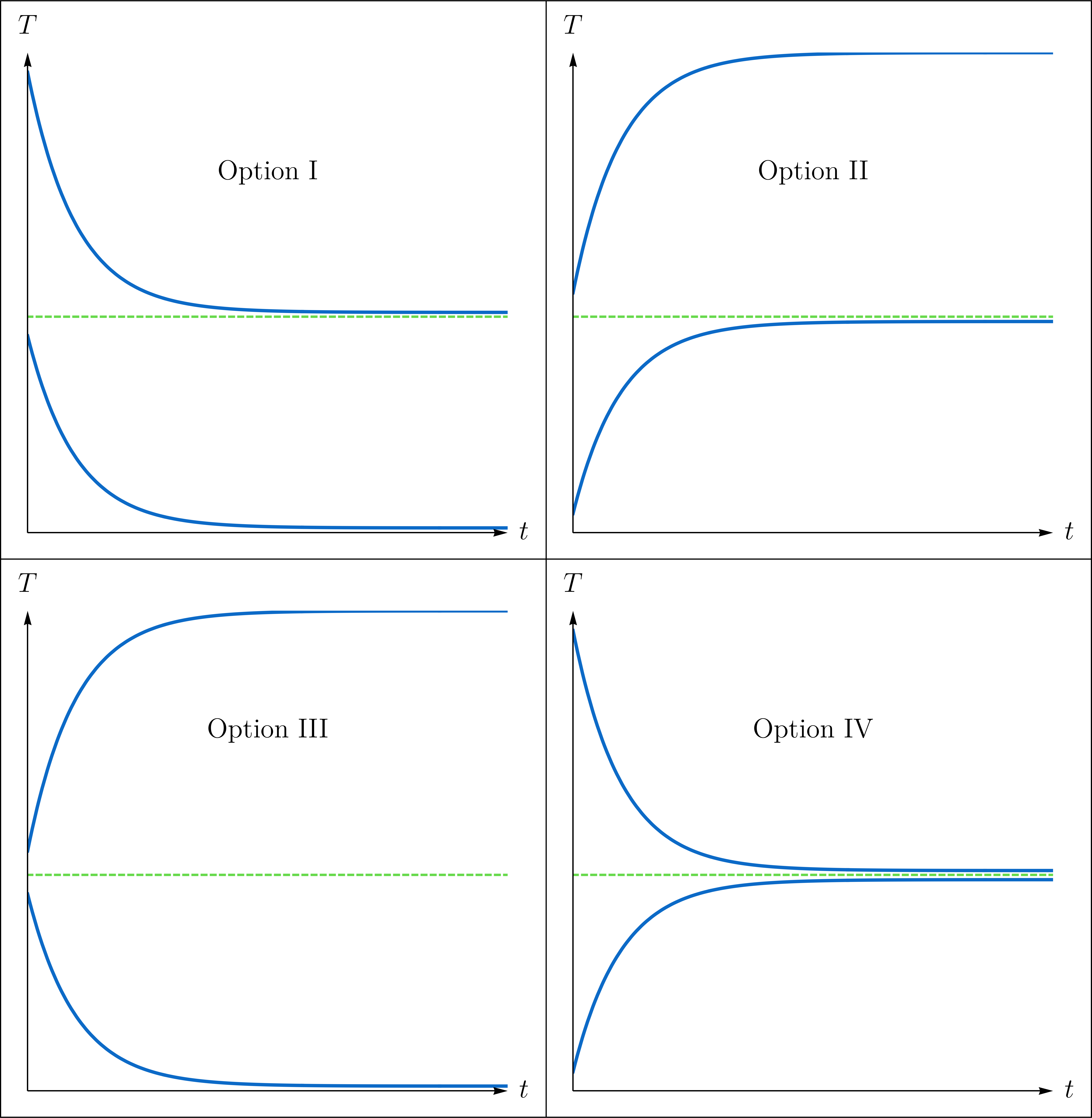Our First Equation

In short, we were able to glean the basic shape of solution curves just from the differential equation $T'(t) = k \big[ T(t) - T_{0}\big]$ by thinking of $T'(t)$ as the rate of change of $T$ at $t$ and using $T''(t)$ to measure the curvature of the graph there.

But there's another way of getting a quick sketch of the solution curves without finding $T(t)$ explicitly. Since $T'(t)$ also gives us the slope of lines tangent to solution curves, we lay down a grid on the $tT$-plane and sketch a short arrow with slope $T'(t_{g})$ at every grid point $(t_{g}, T_{g}).$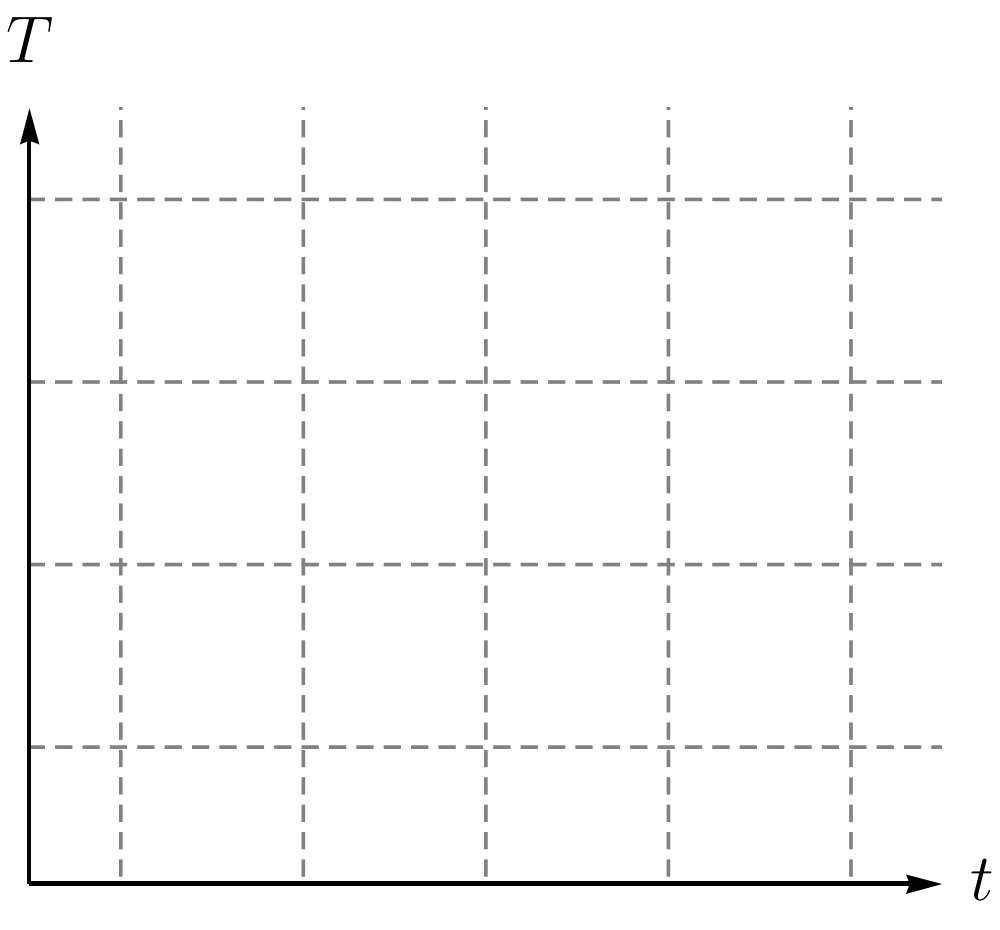This results in a slope or direction field: starting at some point on the $T$-axis, we just follow the arrows to trace the shape of a solution curve with $T(0) = T_{0}.$

The visualization below shows the direction field for our equation with adjustable $T_{\text{air}}$ and $T(0) = T_{0}$ sliders. Notice how the curve follows the arrows for all choices of $T_{0}.$

Our First Equation

We'll solve equations like $T'(t) = k \big[ T(t) - T_{\text{air}}\big]$ later with calculus, but the direction field gives us a foothold for solving our problem right now without integrating. The process we show here will also help us solve systems of first-order equations and higher-order equations.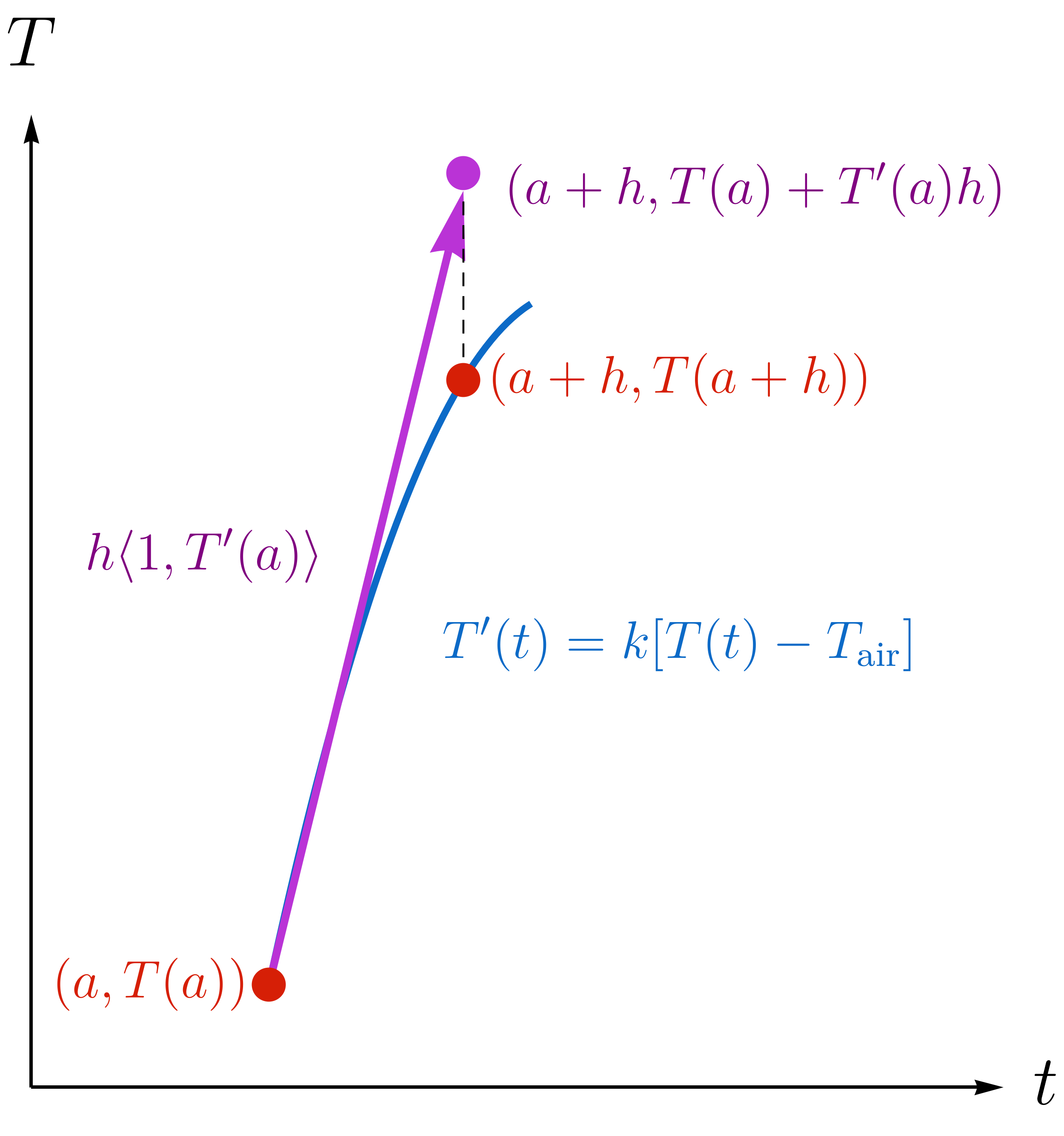Technically, the purple arrows in our direction field are vectors; the components at the point $(t,T)$ in the $tT$-plane are $h \big\langle 1, k [ T-T_{\text{air}} ] \big\rangle,$ where $h > 0.$

We can follow the arrow from $\big(0,T(0)\big)$ to its very tip, which approximates $\big(0+h, T(0+h)\big)$ when $h$ is tiny. At the tip of this vector, we find another direction arrow, which we follow to its tip, and so on. These hops along the arrows give us $T(nh) \approx T_{\text{air}} + [1+ k h ]^{n} \big(T(0)-T_{\text{air}}\big),$ which is an approximation for the temperature at the times $t_{n} = n h, n = 0, 1, 2, 3, \dots$.

The Python codex below computes the first ten approximations and displays the relative error of the approximation for $T(nh)$ compared to the exact result we'll find soon. You can set the hop size $h > 0,$ the initial temperature “To”, and the air temperature “Ta”. We set $k=-1.$

The larger the relative error, the worse the approximation; the smaller the relative error, the better! Play around with different values for the constants and then select the correct option.

import sys
import numpy

h=10;
To=0.5;
Ta=1;
n = 1;

while n <= 10:
c = numpy.absolute(Ta+(To-Ta)*numpy.power(1-numpy.absolute(h),n)-Ta-(To-Ta)*numpy.exp(-n*numpy.absolute(h)))/numpy.absolute(Ta-(To-Ta)*numpy.exp(-n*numpy.absolute(h)))
n = n+1;
print(c)

Choose the size of your hop!

Set your initial temperature!

Set the ambient temperature!

This is our counter; please don't change it!

This measures the percent error of our approximation.

You need to be connected to run code

Our First Equation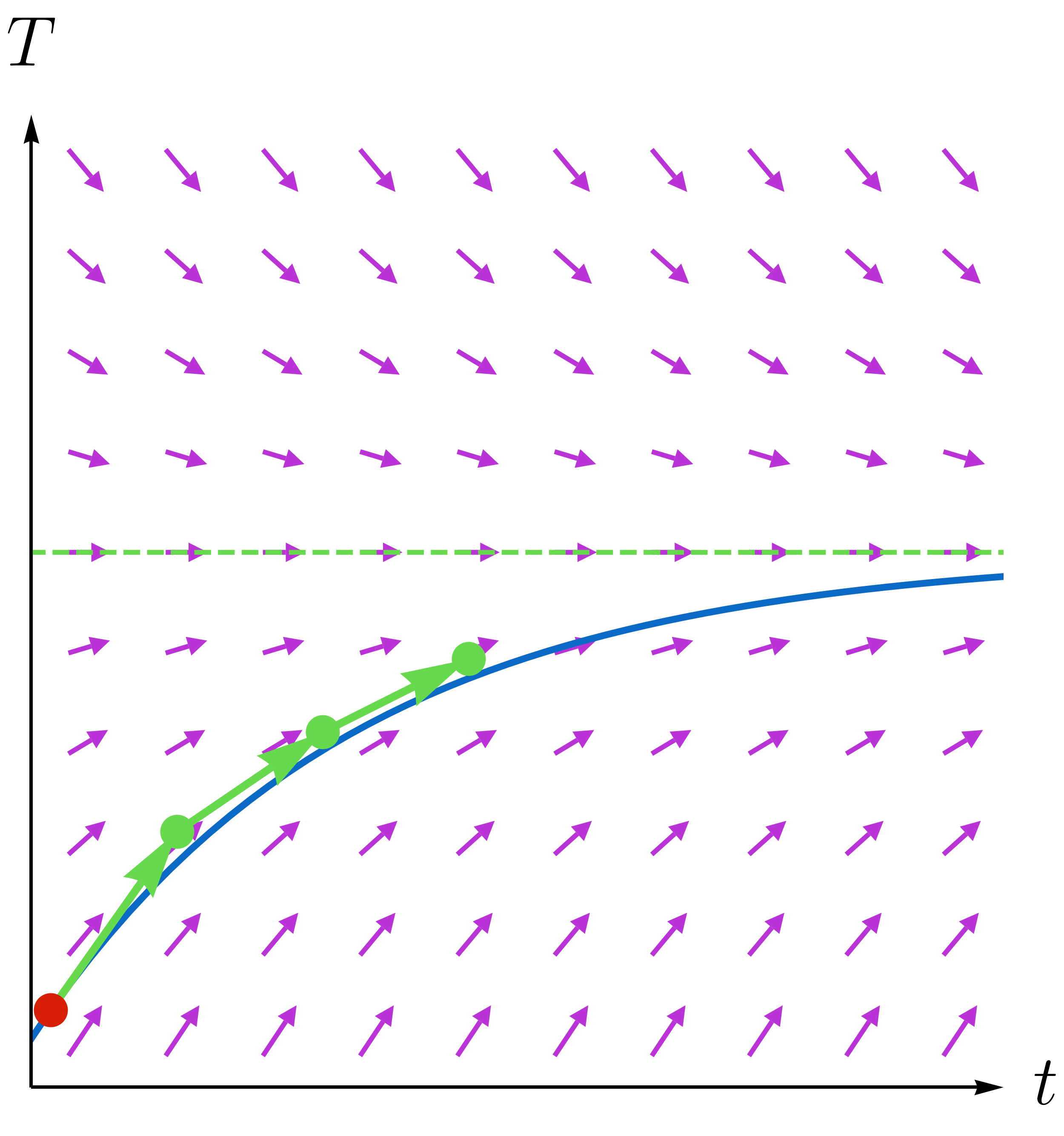The method we just used is attributed to Euler, but beyond this brief shoutout, we won't have very much to say about numerical methods in differential equations. That's a course onto itself!

The main takeaway of the last problem is this: the smaller the value of $h > 0,$ the better the approximation tends to be!

The figure shows the first three approximations we get by successively following the direction field arrows (green) starting from a point (red) on a solution curve (blue) with small hop lengths.

The green points are pretty close to the curve, so this scheme works pretty well. In fact, this process of hopping along direction field arrows is one of the simplest ways of solving a differential equation with a computer, which can be programmed to do the tedious algebra.

We will, however, use this approximation scheme for $T(a+n h)$ to find the exact answer next!

Our First Equation

Challenge Problem:

If we hop along direction field arrows, we come up with the approximation $T(a+nh) \approx T_{\text{air}} + [1+ k h ]^{n} \big(T(a)-T_{\text{air}}\big),$ which we used in Euler's method a few pages back.

If we want to estimate the solution starting at the value $T_{0},$ we should set $T(0) = T_{0}$ and take $T(0+nh) =T(nh), n = 1, 2, \dots$ as our approximation for the curve with $T(0) = T_{0}:$ $T(nh) \approx T_{\text{air}} + [1+ k h ]^{n} (T_{0}-T_{\text{air}}).$ To estimate $T(t)$ where $t > 0$ is a fixed number, set $t = n h$ and write $T(t) \approx T_{\text{air}} + \bigg[1+ k \underbrace{ \left(\frac{t}{n} \right)}_{ h} \bigg]^{n} (T_{0}-T_{\text{air}}).$ This becomes exact if we take the limit as $h \to 0,$ which is equivalent to taking $n \to \infty.$ At least, this is our expectation based on our experiments with Euler's method!

What is the result of this limit and therefore the exact value of $T(t) ?$

Good to know: Euler's number is defined by $e = \lim\limits_{x \to \infty} \left( 1+ \frac{1}{x} \right)^{x}.$

Our First Equation

From this brief unit, we get the gist of differential equations, but the topic is vast, and we have a long road ahead of us. While we aim to be as self-contained as possible, some parts of our course rely heavily on results and techniques in other areas: no math is an island entire of itself!

Below is a guide to some of the more important topics and where they pop up in our course:

The next unit is a collection of exercises designed to help to refresh your memory on some of the topics described here. It stands outside the mainstream of our narrative.

Our First Equation

×